Warning: count(): Parameter must be an array or an object that implements Countable in /home/customer/www/thegatebook.in/public_html/qa/qa-include/qa-theme-base.php on line 177

# DSA - Graphs and Hashing -Q10

Warning: count(): Parameter must be an array or an object that implements Countable in /home/customer/www/thegatebook.in/public_html/qa/qa-include/qa-theme-base.php on line 177

Professor Borden proposes a new divide and conquer algorithm for computing minimum spanning trees, which goes as follows. Given a graph G = (V,E), partition the set V of vertices into two sets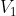and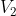such that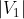and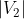differ by atmost 1. Let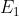be the set of edges that are incident only on vertices in, and let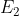be the set of edges that are incident only on vertices in. Recursively solve a minimum-spanning-tree problem on each of the two subgraphs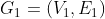and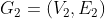. Finally select the minimum weight edge in E that crosses the cut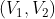and use this edge to unite the resulting two minimum spanning trees into single spanning tree.

Which one of the following statements is true ?

(A)The algorithm computes minimum spanning trees for some graphs but not for all

(B)The algorithm computes minimum spanning trees for all the graphs

(C)The algorithm fails in computing  minimum spanning trees for all the graphs.

(D)The algorithm works correctly in computing  minimum spanning trees for all the graphs with distinct edge weights.asked Aug 3, 2020
reshown Aug 6, 2020

+1 vote

We claim that the algorithm will fail. A simple counter example is shown in Figure 1. Graph G = (V, E) has four vertices: {v1, v2, v3, v4}, and is partitioned into subsets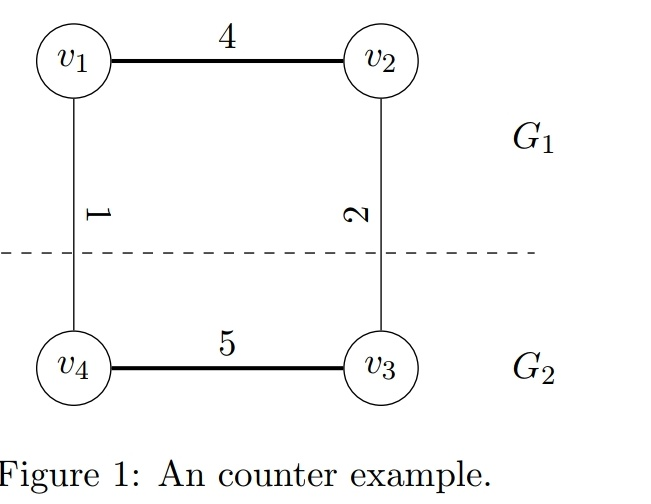G1 with V1 = {v1, v2} and G2 with V2 = {v3, v4}. The minimum spanning tree(MST) of G1 has weight 4, and the MST of G2 has weight 5, and the minimum-weight edge crossing thec cut(V1, V2) has weight 1, in sum the spanning tree forming by the proposed algorithm is v2 − v1 − v4 − v3 which has weight 10. On the contrary, it is obvious that the MST of G is v4 − v1 − v2 − v3 with weight 7. Hence the proposed algorithm fails to obtain an MST.

btw it is clrs question, check last question
https://github.com/gzc/CLRS/blob/master/C23-Minimum-Spanning-Trees/23.2.mdanswered Aug 14, 2020 by (226,240 points)
Thanks Deepak